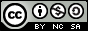- 目录 -

# 常量指针和指针常量和常量指针常量

 1 2  int n = 10; const int *p = &n; 

 1 2 3 4  int n = 10, m = 30; const int *p = &n;//常量指针p初始化指向n *p = 20;// 这一步错误，这是一个常量指针，不能更改目标数据对象的值 p = &m;//这一步正确，可以修改指向 

 1  void PrintObj(const int *p); 

 1 2 3 4  int n = 10, m = 30; int *const p = &n;//定义一个指针常量指向n *p = 40;//合法，可以改变指针所指的目标数据对象的值 p = &m;//不合法，不能修改指针指向。 

 1 2 3 4  int n = 10, m = 30; const int *const p = &n; *p = 40;//错误 p = &m;//错误